# Ultraspherical polynomials

(diff) ← Older revision | Latest revision (diff) | Newer revision → (diff)

Gegenbauer polynomials

Orthogonal polynomials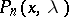on the interval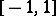with the weight function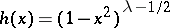; a particular case of the Jacobi polynomials for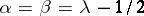(); the Legendre polynomials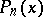are a particular case of the ultraspherical polynomials: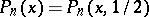.

For ultraspherical polynomials one has the standardizationand the representation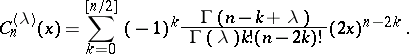The ultraspherical polynomials are the coefficients of the power series expansion of the generating functionThe ultraspherical polynomialsatisfies the differential equation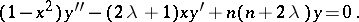More commonly used are the formulas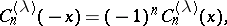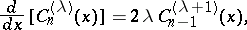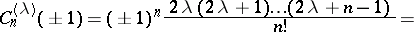For references see Orthogonal polynomials.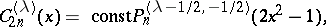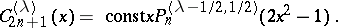See [a1] for-ultraspherical polynomials.